Sutda Game
Check the rules of the game, fill in the functions below, select your opponent and press the start button to start the game.
You and your opponent will each receive 2 cards (Hwatu card), and it is a game where you win or lose with a combination of 2 cards.
There are 20 cards in total, and there are two pairs of cards that represent the numbers 1 to 10. It is assumed that the cards consist of numbers 1 to 10 and numbers 11 to 20 so that only the numbers can be distinguished. For example, 15 is the second card meaning 5.
You get money when the game starts, you get 2 cards and 1 opponent card at the start of the round, and you have to choose raise or call or die on each turn.
"raise" is an action that raises table money, "call" is an action that accepts only the amount of money raised by the opponent and ends the round and confirms the win or lose, and "die" is an action that gives up the opponent's card check and pays only the basic money(1) before giving up the round.
Code battle is a battle between your code and opponent's code, and the language is JAVASCRIPT.
Fill in the code, select the opponent (LEVEL1, LEVEL2, LEVEL3, Online opponent) and then press the game start button will start the match.
1 game consists of a maximum of 200 rounds and the game ends if either you or your opponent runs out of money while the round is repeated. And, 1 round consists of several turns. On each turn, you can "raise" to raise table money and pass it to your opponent's turn, or you can "call" or "die" to finish the round.
OnGameStart and onGameEnd functions are called at the beginning and end of the game, onRoundStart and onRoundEnd functions are called at the beginning and end of each round, and onTurnStart is called on each turn. onGameStart => onRoundStart => onTurnStart => onRoundEnd => onGameEnd. You can implement your own logic in each function.
Building a basic strategy of the game, analyzing your opponent's pattern and returning the best choice from onTurnStart is the key to winning.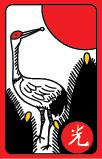1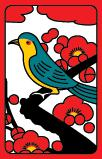23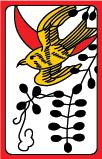45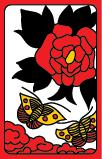67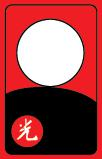8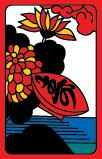9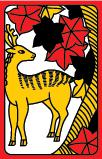10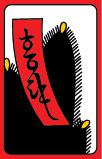111213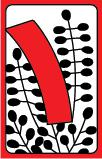14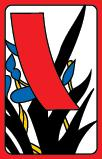15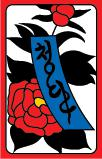16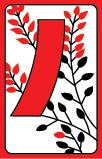17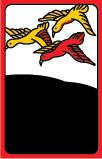18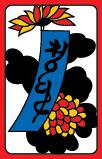19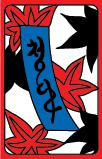20
OrderGroupNameRemarks
1Gwang-ttaeng3 and 8 gwang-ttaengWhen two cards are 3 and 8. Sutda's strongest combination.
2Gwang-ttaeng1 and 8 gwang-ttaengWhen two cards are 1 and 8.
3Gwang-ttaeng1 and 3 gwang-ttaengWhen two cards are 1 and 3.
4Ttaeng10 ttaengWhen two cards are 10 and 20.
5Ttaeng9 ttaengWhen two cards are 9 and 19.
6Ttaeng8 ttaengWhen two cards are 8 and 18.
7Ttaeng7 ttaengWhen two cards are 7 and 17.
8Ttaeng6 ttaengWhen two cards are 6 and 16.
9Ttaeng5 ttaengWhen two cards are 5 and 15.
10Ttaeng4 ttaengWhen two cards are 4 and 14.
11Ttaeng3 ttaengWhen two cards are 3 and 13.
12Ttaeng2 ttaengWhen two cards are 2 and 12.
13Ttaeng1 ttaengWhen two cards are 1 and 11.
14Kkeus9 kkeusWhen the "units" number of the sum of two card is 9. (Ex: 5 and 14, or 3 and 6). If it's the same, compare the big cards. (Ex: ['5' and 4] < [3 and '6'])
15Kkeus8 kkeusWhen the "units" number of the sum of two card is 8. (Ex: 5 and 13, or 6 and 2)
16Kkeus7 kkeusWhen the "units" number of the sum of two card is 7. (Ex: 2 and 15, or 3 and 4)
17Kkeus6 kkeusWhen the "units" number of the sum of two card is 6. (Ex: 5 and 11, or 1 and 5)
18Kkeus5 kkeusWhen the "units" number of the sum of two card is 5. (Ex: 2 and 13, or 3 and 2)
19Kkeus4 kkeusWhen the "units" number of the sum of two card is 4. (Ex: 4 and 10, or 1 and 3)
20Kkeus3 kkeusWhen the "units" number of the sum of two card is 3. (Ex: 1 and 12, or 2 and 1)
21Kkeus2 kkeusWhen the "units" number of the sum of two card is 2. (Ex: 2 and 10, or 3 and 9)
22Kkeus1 kkeusWhen the "units" number of the sum of two card is 1. (Ex: 1 and 10, or 5 and 6)
23Kkeus0 kkeusWhen the "units" number of the sum of two card is 0. (Ex: 1 and 9, or 3 and 7)
Opponents: <= If you join here, other users can see you.
 ME TURN OP raise or call or die raise or call or dieROUND ME OP 0+0 0+0
 GAME WIN LOSE 0 0
The entire game log is displayed in the area below.
If you or the opponent's screen is not visible, the communication speed will be slower.